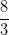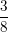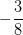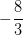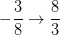SSAT Upper Level Math : Lines

Example Questions

Example Question #7 : How To Find The Slope Of A Perpendicular Line

A line is defined by the following equation: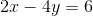What is the slope of a line that is perpendicular to the line above?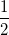Explanation:

The equation of a line iswhereis the slope.

Rearrange the equation to match this: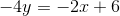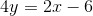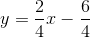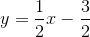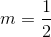For the perpendicular line, the slope is the negative reciprocal;

therefore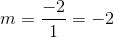Example Question #2 : How To Find The Slope Of Perpendicular Lines

Find the slope of the line perpendicular to the line that has the equation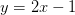.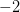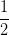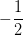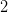Explanation:

Perpendicular lines have slopes that are negative reciprocals of each other. In other words, change the sign and flip the fraction around.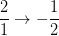Example Question #3 : How To Find The Slope Of Perpendicular Lines

Find the slope of a line perpendicular to the line with the equation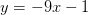.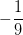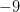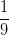Explanation:

Perpendicular lines have slopes that are negative reciprocals of each other. In other words, change the sign and flip the fraction around to find the slope of the line perpendicular to the given one.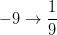Example Question #71 : Coordinate Geometry

Find the slope of a line that is perpendicular to the line with the equation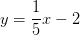.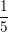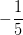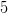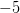Explanation:

Perpendicular lines have slopes that are negative reciprocals of each other. In other words, change the sign and flip the fraction around to find the slope of the line perpendicular to the given one.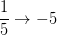Example Question #72 : Coordinate Geometry

Find the slope of a line that is perpendicular to the line with the equation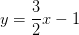.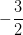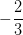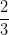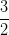Explanation:

Perpendicular lines have slopes that are negative reciprocals of each other. In other words, change the sign and flip the fraction around to find the slope of the line perpendicular to the given one.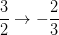Example Question #73 : Coordinate Geometry

Find the slope of a line that is perpendicular to the line with the equation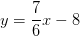.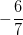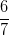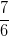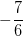Explanation:

Perpendicular lines have slopes that are negative reciprocals of each other. In other words, change the sign and flip the fraction around to find the slope of the line perpendicular to the given one.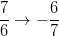Example Question #74 : Coordinate Geometry

Find the slope of a line that is perpendicular to the line with the equation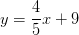.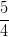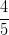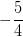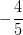Explanation:

Perpendicular lines have slopes that are negative reciprocals of each other. In other words, change the sign and flip the fraction around to find the slope of the line perpendicular to the given one.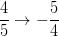Example Question #75 : Coordinate Geometry

Find the slope of a line that is perpendicular to the line with the equation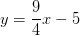.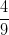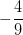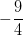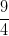Explanation:

Perpendicular lines have slopes that are negative reciprocals of each other. In other words, change the sign and flip the fraction around to find the slope of the line perpendicular to the given one.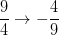Example Question #76 : Coordinate Geometry

Find the slope of a line that is perpendicular to the line with the equation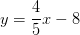.Explanation:

Perpendicular lines have slopes that are negative reciprocals of each other. In other words, change the sign and flip the fraction around to find the slope of the line perpendicular to the given one.Example Question #77 : Coordinate Geometry

Find the slope of a line that is perpendicular to the line with the equation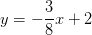.